# An introduction to property based testing

Jun. 10, 2015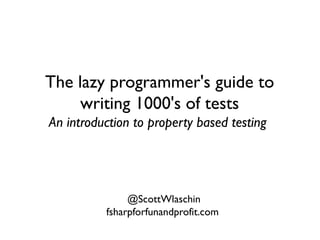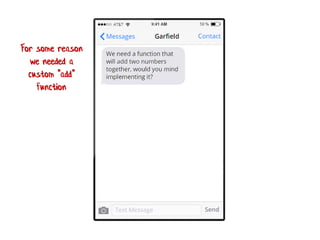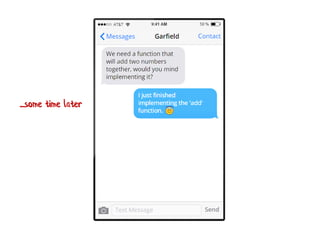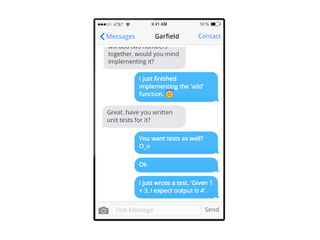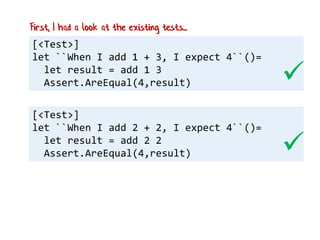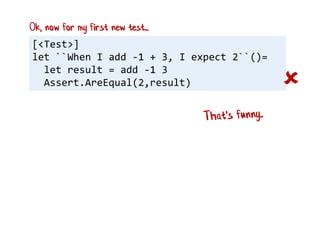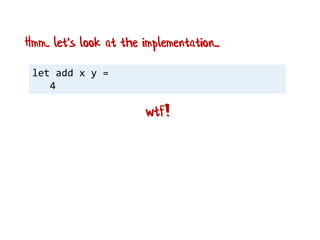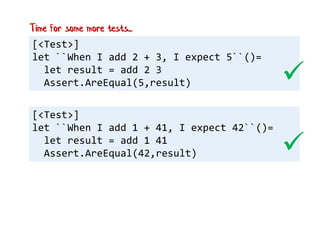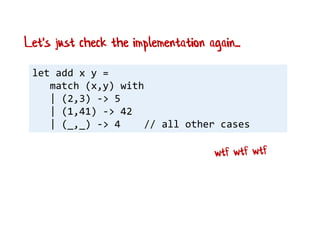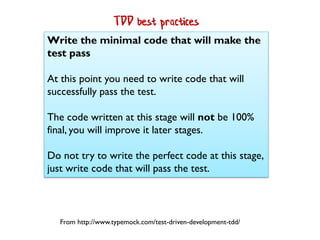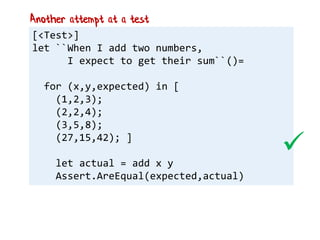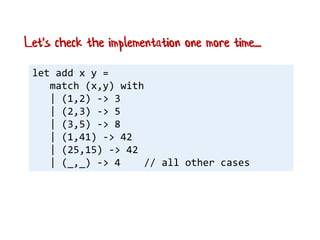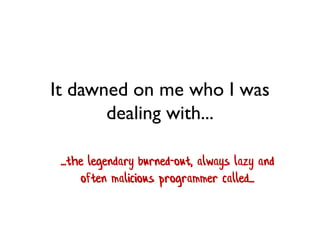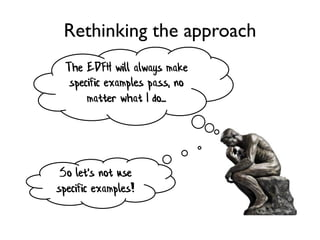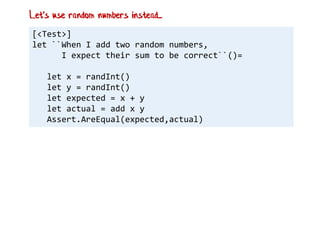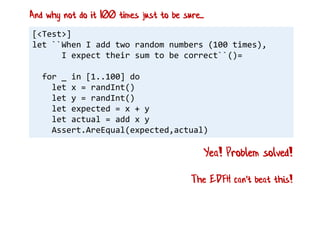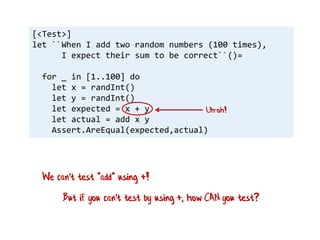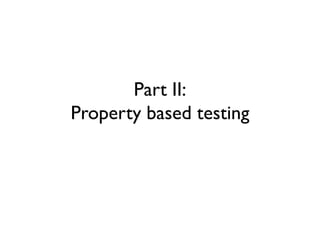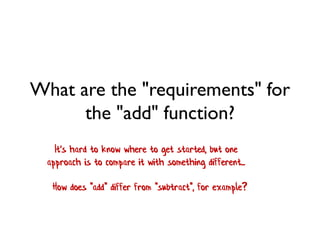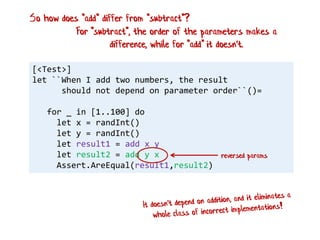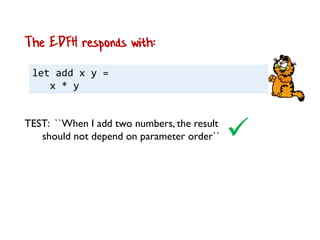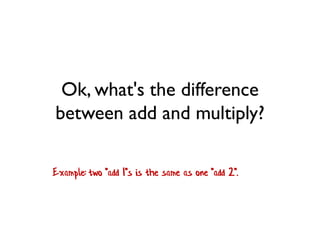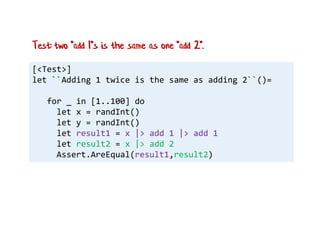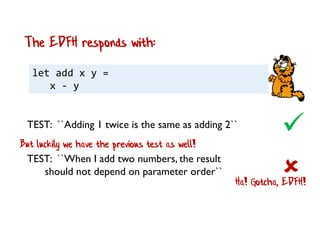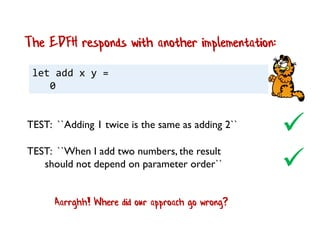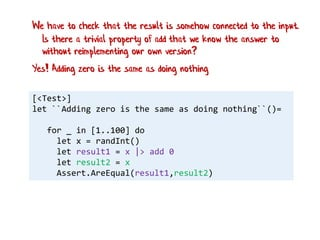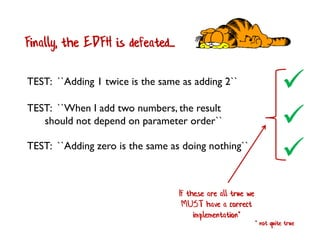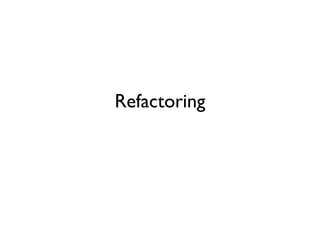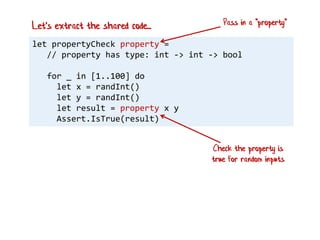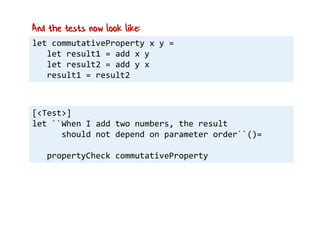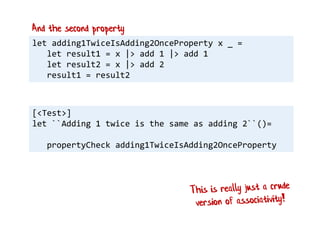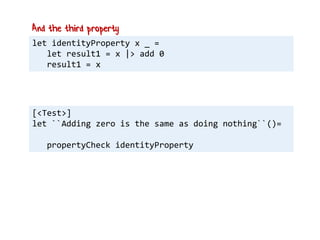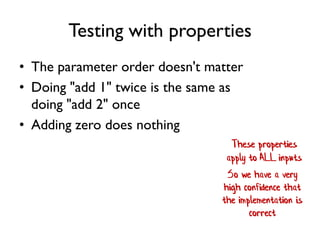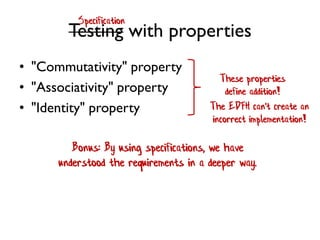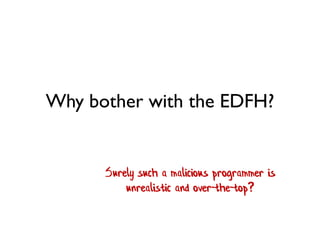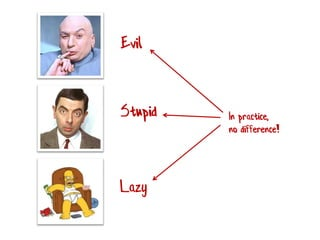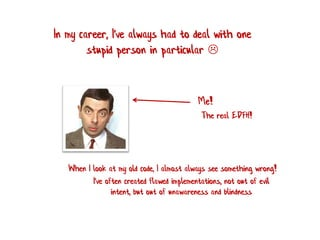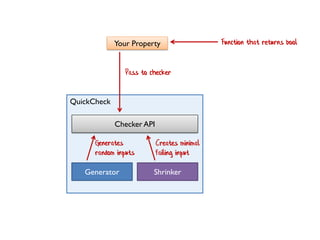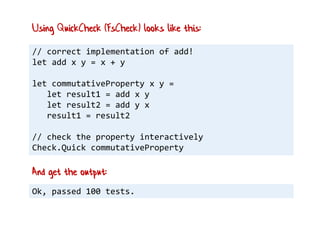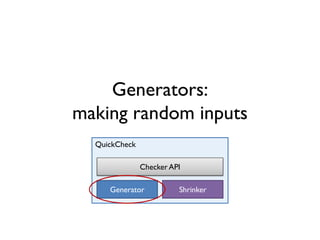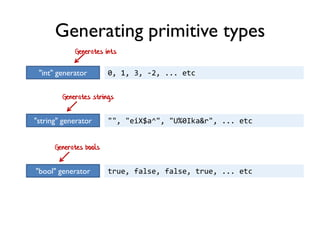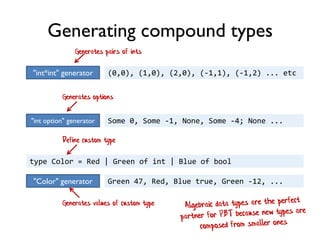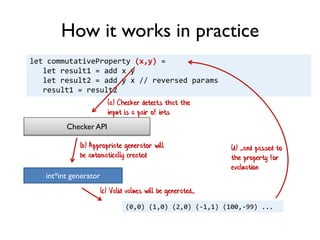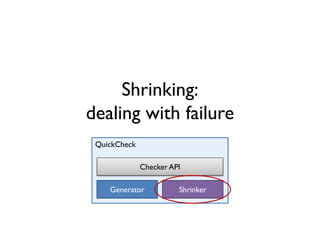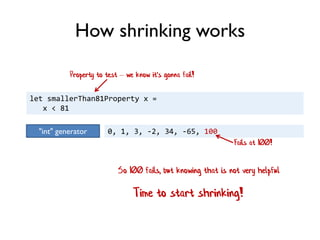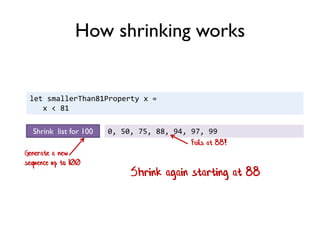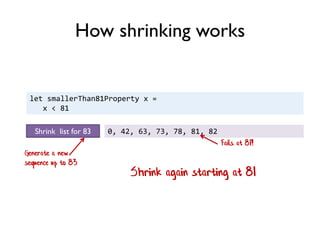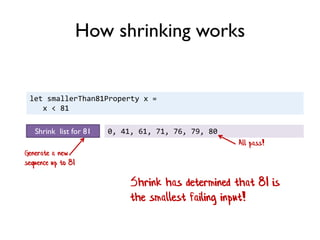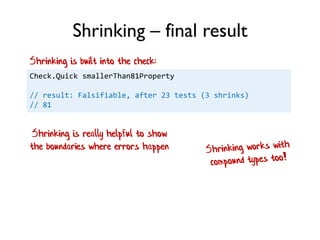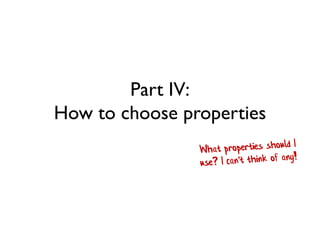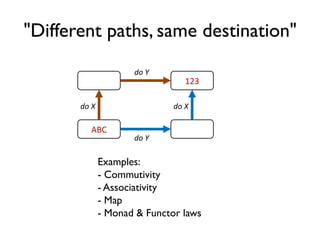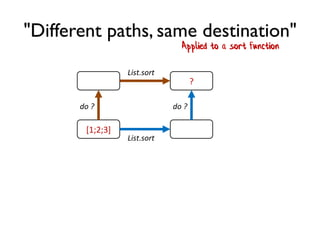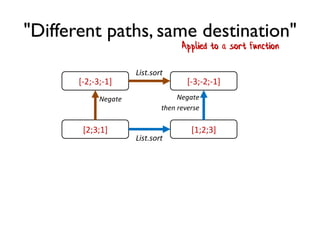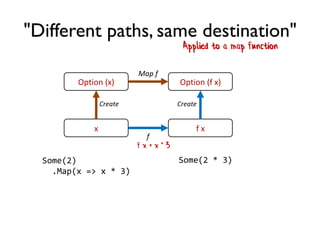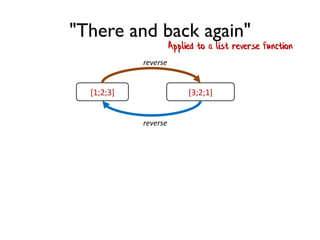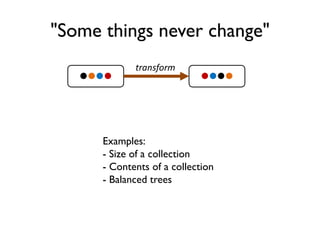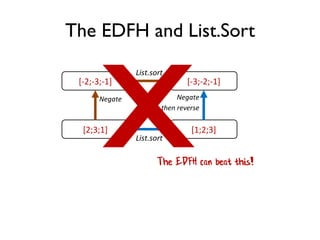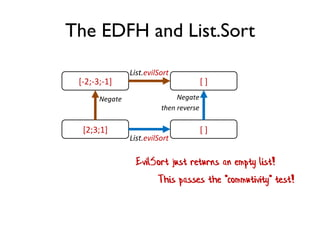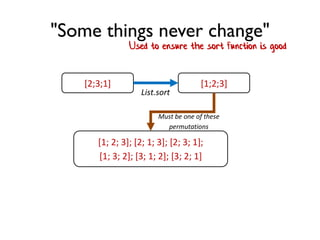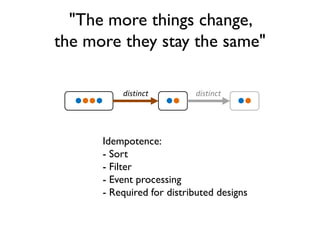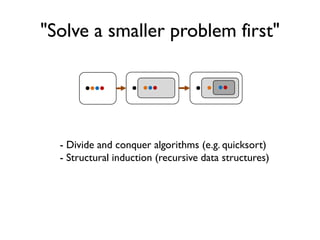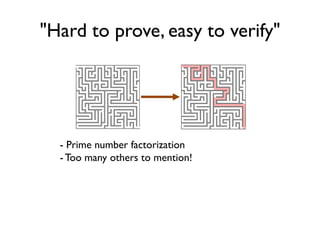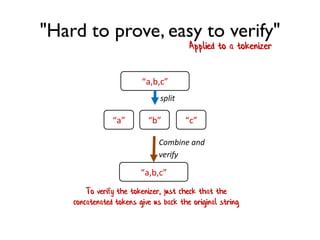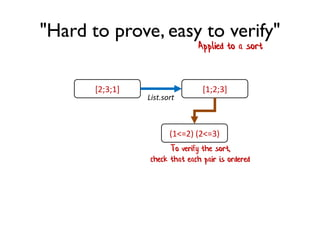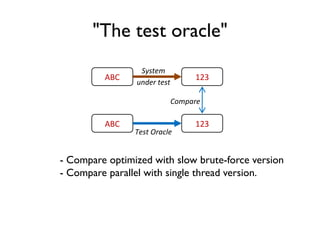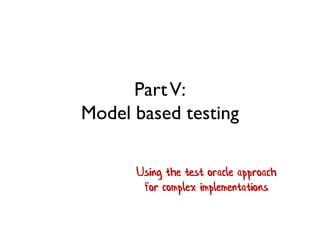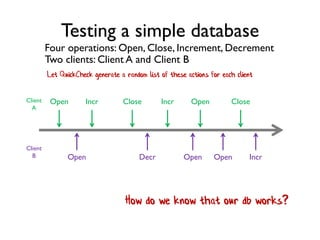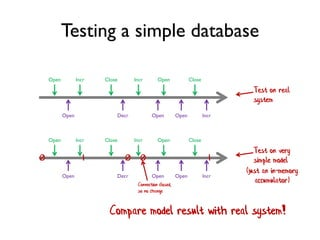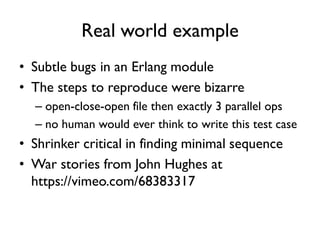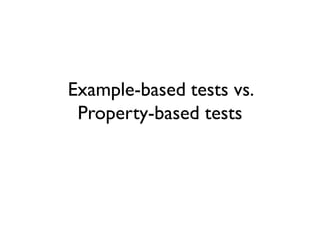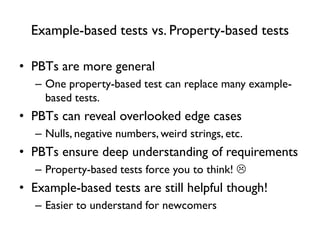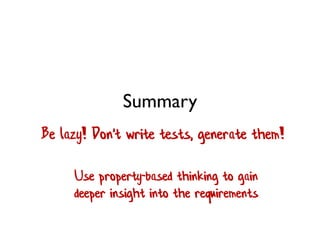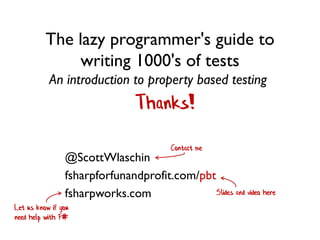1 of 97

### An introduction to property based testing

• 1. The lazy programmer's guide to writing 1000's of tests An introduction to property based testing @ScottWlaschin fsharpforfunandprofit.com
• 2. Part 1: In which I have a conversation with a remote developer This was a project from a long time ago, in a galaxy far far away
• 3. For some reason we needed a custom "add" function
• 17. So I decide to start writing the unit tests myself
• 18. [<Test>] let ``When I add 1 + 3, I expect 4``()= let result = add 1 3 Assert.AreEqual(4,result) [<Test>] let ``When I add 2 + 2, I expect 4``()= let result = add 2 2 Assert.AreEqual(4,result)   First, I had a look at the existing tests...
• 19. [<Test>] let ``When I add -1 + 3, I expect 2``()= let result = add -1 3 Assert.AreEqual(2,result)  Ok, now for my first new test...
• 20. let add x y = 4 wtf! Hmm.. let's look at the implementation...
• 24. [<Test>] let ``When I add 2 + 3, I expect 5``()= let result = add 2 3 Assert.AreEqual(5,result) [<Test>] let ``When I add 1 + 41, I expect 42``()= let result = add 1 41 Assert.AreEqual(42,result)   Time for some more tests...
• 25. let add x y = match (x,y) with | (2,3) -> 5 | (1,41) -> 42 | (_,_) -> 4 // all other cases Let's just check the implementation again...
• 29. Write the minimal code that will make the test pass At this point you need to write code that will successfully pass the test. The code written at this stage will not be 100% final, you will improve it later stages. Do not try to write the perfect code at this stage, just write code that will pass the test. From http://www.typemock.com/test-driven-development-tdd/ TDD best practices
• 30. [<Test>] let ``When I add two numbers, I expect to get their sum``()= for (x,y,expected) in [ (1,2,3); (2,2,4); (3,5,8); (27,15,42); ] let actual = add x y Assert.AreEqual(expected,actual) Another attempt at a test 
• 31. let add x y = match (x,y) with | (1,2) -> 3 | (2,3) -> 5 | (3,5) -> 8 | (1,41) -> 42 | (25,15) -> 42 | (_,_) -> 4 // all other cases Let's check the implementation one more time....
• 32. It dawned on me who I was dealing with... ...the legendary burned-out, always lazy and often malicious programmer called...
• 34. Rethinking the approach The EDFH will always make specific examples pass, no matter what I do... So let's not use specific examples!
• 35. [<Test>] let ``When I add two random numbers, I expect their sum to be correct``()= let x = randInt() let y = randInt() let expected = x + y let actual = add x y Assert.AreEqual(expected,actual) Let's use random numbers instead...
• 36. [<Test>] let ``When I add two random numbers (100 times), I expect their sum to be correct``()= for _ in [1..100] do let x = randInt() let y = randInt() let expected = x + y let actual = add x y Assert.AreEqual(expected,actual) Yea! Problem solved! And why not do it 100 times just to be sure... The EDFH can't beat this!
• 37. [<Test>] let ``When I add two random numbers (100 times), I expect their sum to be correct``()= for _ in [1..100] do let x = randInt() let y = randInt() let expected = x + y let actual = add x y Assert.AreEqual(expected,actual) Uh-oh! But if you can't test by using +, how CAN you test? We can't test "add" using +!
• 39. What are the "requirements" for the "add" function? It's hard to know where to get started, but one approach is to compare it with something different... How does "add" differ from "subtract", for example?
• 40. [<Test>] let ``When I add two numbers, the result should not depend on parameter order``()= for _ in [1..100] do let x = randInt() let y = randInt() let result1 = add x y let result2 = add y x Assert.AreEqual(result1,result2) reversed params So how does "add" differ from "subtract"? For "subtract", the order of the parameters makes a difference, while for "add" it doesn't.
• 41. let add x y = x * y The EDFH responds with: TEST: ``When I add two numbers, the result should not depend on parameter order``
• 42. Ok, what's the difference between add and multiply? Example: two "add 1"s is the same as one "add 2".
• 43. [<Test>] let ``Adding 1 twice is the same as adding 2``()= for _ in [1..100] do let x = randInt() let y = randInt() let result1 = x |> add 1 |> add 1 let result2 = x |> add 2 Assert.AreEqual(result1,result2) Test: two "add 1"s is the same as one "add 2".
• 44. let add x y = x - y The EDFH responds with:  TEST: ``When I add two numbers, the result should not depend on parameter order`` TEST: ``Adding 1 twice is the same as adding 2`` Ha! Gotcha, EDFH! But luckily we have the previous test as well!
• 45. let add x y = 0 The EDFH responds with another implementation:  TEST: ``When I add two numbers, the result should not depend on parameter order`` TEST: ``Adding 1 twice is the same as adding 2``  Aarrghh! Where did our approach go wrong?
• 46. [<Test>] let ``Adding zero is the same as doing nothing``()= for _ in [1..100] do let x = randInt() let result1 = x |> add 0 let result2 = x Assert.AreEqual(result1,result2) Yes! Adding zero is the same as doing nothing We have to check that the result is somehow connected to the input. Is there a trivial property of add that we know the answer to without reimplementing our own version?
• 47. Finally, the EDFH is defeated...  TEST: ``When I add two numbers, the result should not depend on parameter order`` TEST: ``Adding 1 twice is the same as adding 2``  TEST: ``Adding zero is the same as doing nothing``  If these are all true we MUST have a correct implementation* * not quite true
• 49. let propertyCheck property = // property has type: int -> int -> bool for _ in [1..100] do let x = randInt() let y = randInt() let result = property x y Assert.IsTrue(result) Let's extract the shared code... Pass in a "property" Check the property is true for random inputs
• 50. let commutativeProperty x y = let result1 = add x y let result2 = add y x result1 = result2 And the tests now look like: [<Test>] let ``When I add two numbers, the result should not depend on parameter order``()= propertyCheck commutativeProperty
• 52. let identityProperty x _ = let result1 = x |> add 0 result1 = x And the third property [<Test>] let ``Adding zero is the same as doing nothing``()= propertyCheck identityProperty
• 54. Testing with properties • The parameter order doesn't matter • Doing "add 1" twice is the same as doing "add 2" once • Adding zero does nothing These properties apply to ALL inputs So we have a very high confidence that the implementation is correct
• 55. Testing with properties • "Commutativity" property • "Associativity" property • "Identity" property These properties define addition! The EDFH can't create an incorrect implementation! Bonus: By using specifications, we have understood the requirements in a deeper way. Specification
• 56. Why bother with the EDFH? Surely such a malicious programmer is unrealistic and over-the-top?
• 58. In my career, I've always had to deal with one stupid person in particular  Me! When I look at my old code, I almost always see something wrong! I've often created flawed implementations, not out of evil intent, but out of unawareness and blindness The real EDFH!
• 59. Part III: QuickCheck and its ilk Wouldn't it be nice to have a toolkit for doing this? The "QuickCheck" library was originally developed for Haskell by Koen Claessen and John Hughes, and has been ported to many other languages.
• 60. QuickCheck Generator Shrinker Your Property Function that returns bool Checker API Pass to checker Generates random inputs Creates minimal failing input
• 61. // correct implementation of add! let add x y = x + y let commutativeProperty x y = let result1 = add x y let result2 = add y x result1 = result2 // check the property interactively Check.Quick commutativeProperty Using QuickCheck (FsCheck) looks like this: Ok, passed 100 tests. And get the output:
• 63. Generates ints "int" generator 0, 1, 3, -2, ... etc Generates strings "string" generator "", "eiX\$a^", "U%0Ika&r", ... etc "bool" generator true, false, false, true, ... etc Generating primitive types Generates bools
• 64. Generates pairs of ints "int*int" generator (0,0), (1,0), (2,0), (-1,1), (-1,2) ... etc Generates options "int option" generator Some 0, Some -1, None, Some -4; None ... "Color" generator Green 47, Red, Blue true, Green -12, ... Generating compound types type Color = Red | Green of int | Blue of bool Generates values of custom type Define custom type
• 65. let commutativeProperty (x,y) = let result1 = add x y let result2 = add y x // reversed params result1 = result2 (b) Appropriate generator will be automatically created int*int generator (0,0) (1,0) (2,0) (-1,1) (100,-99) ... (a) Checker detects that the input is a pair of ints Checker API (c) Valid values will be generated... (d) ...and passed to the property for evaluation How it works in practice
• 67. let smallerThan81Property x = x < 81 Property to test – we know it's gonna fail! "int" generator 0, 1, 3, -2, 34, -65, 100 Fails at 100! So 100 fails, but knowing that is not very helpful How shrinking works Time to start shrinking!
• 68. let smallerThan81Property x = x < 81 Shrink again starting at 88 How shrinking works Shrink list for 100 0, 50, 75, 88, 94, 97, 99 Fails at 88! Generate a new sequence up to 100
• 69. let smallerThan81Property x = x < 81 Shrink again starting at 83 How shrinking works Shrink list for 88 0, 44, 66, 77, 83, 86, 87 Fails at 83! Generate a new sequence up to 88
• 70. let smallerThan81Property x = x < 81 Shrink again starting at 81 How shrinking works Shrink list for 83 0, 42, 63, 73, 78, 81, 82 Fails at 81! Generate a new sequence up to 83
• 71. let smallerThan81Property x = x < 81 Shrink has determined that 81 is the smallest failing input! How shrinking works Shrink list for 81 0, 41, 61, 71, 76, 79, 80 All pass! Generate a new sequence up to 81
• 72. Shrinking – final result Check.Quick smallerThan81Property // result: Falsifiable, after 23 tests (3 shrinks) // 81 Shrinking is really helpful to show the boundaries where errors happen Shrinking is built into the check:
• 73. Part IV: How to choose properties
• 74. ABC 123 do X do X do Y do Y "Different paths, same destination" Examples: - Commutivity - Associativity - Map - Monad & Functor laws
• 75. "Different paths, same destination" Applied to a sort function [1;2;3] ? do ? do ? List.sort List.sort
• 76. "Different paths, same destination" Applied to a sort function [2;3;1] [-2;-3;-1] [-3;-2;-1] [1;2;3] Negate List.sort List.sort Negate then reverse
• 77. "Different paths, same destination" Applied to a map function Some(2) .Map(x => x * 3) Some(2 * 3) x Option (x) Option (f x) f x Create Map f f Create f x = x * 3
• 78. "There and back again" ABC 100101001 Do X Inverse Examples: - Serialization/Deserialization - Addition/Subtraction -Write/Read - SetProperty/GetProperty
• 79. "There and back again" Applied to a list reverse function [1;2;3] [3;2;1] reverse reverse
• 80. "Some things never change"   transform Examples: - Size of a collection - Contents of a collection - Balanced trees
• 82. The EDFH and List.Sort [2;3;1] [-2;-3;-1] [ ] [ ] Negate List.evilSort List.evilSort Negate then reverse EvilSort just returns an empty list! This passes the "commutivity" test!
• 83. "Some things never change" [2;3;1] [1; 2; 3]; [2; 1; 3]; [2; 3; 1]; [1; 3; 2]; [3; 1; 2]; [3; 2; 1] [1;2;3] List.sort Must be one of these permutations Used to ensure the sort function is good
• 84. "The more things change, the more they stay the same"   distinct  distinct Idempotence: - Sort - Filter - Event processing - Required for distributed designs
• 85. "Solve a smaller problem first"       - Divide and conquer algorithms (e.g. quicksort) - Structural induction (recursive data structures)
• 86. "Hard to prove, easy to verify" - Prime number factorization -Too many others to mention!
• 87. "Hard to prove, easy to verify" Applied to a tokenizer “a,b,c” split “a” “b” “c” “a,b,c” Combine and verify To verify the tokenizer, just check that the concatenated tokens give us back the original string
• 88. "Hard to prove, easy to verify" Applied to a sort To verify the sort, check that each pair is ordered [2;3;1] (1<=2) (2<=3) [1;2;3] List.sort
• 89. ABC ABC 123 123 Compare System under test Test Oracle "The test oracle" - Compare optimized with slow brute-force version - Compare parallel with single thread version.
• 90. PartV: Model based testing Using the test oracle approach for complex implementations
• 91. Testing a simple database Open Incr Close Incr Open Close Open Decr Open Four operations: Open, Close, Increment, Decrement How do we know that our db works? Let QuickCheck generate a random list of these actions for each client Open Incr Client A Client B Two clients: Client A and Client B
• 92. Testing a simple database Compare model result with real system! Open Incr Close Incr Open Close Open Decr Open Open Incr Test on real system Open Incr Close Incr Open Close Open Decr Open Open Incr Test on very simple model1 00 0 1 (just an in-memory accumulator)Connection closed, so no change
• 93. Real world example • Subtle bugs in an Erlang module • The steps to reproduce were bizarre – open-close-open file then exactly 3 parallel ops – no human would ever think to write this test case • Shrinker critical in finding minimal sequence • War stories from John Hughes at https://vimeo.com/68383317
• 95. Example-based tests vs. Property-based tests • PBTs are more general – One property-based test can replace many example- based tests. • PBTs can reveal overlooked edge cases – Nulls, negative numbers, weird strings, etc. • PBTs ensure deep understanding of requirements – Property-based tests force you to think!  • Example-based tests are still helpful though! – Easier to understand for newcomers
• 96. Summary Be lazy! Don't write tests, generate them! Use property-based thinking to gain deeper insight into the requirements
• 97. The lazy programmer's guide to writing 1000's of tests An introduction to property based testing Let us know if you need help with F# Thanks! @ScottWlaschin fsharpforfunandprofit.com/pbt fsharpworks.com Slides and video here Contact me